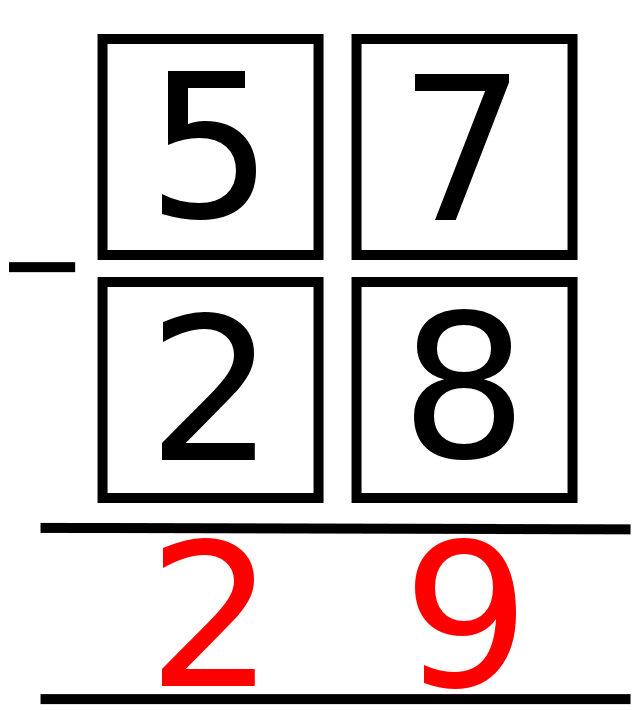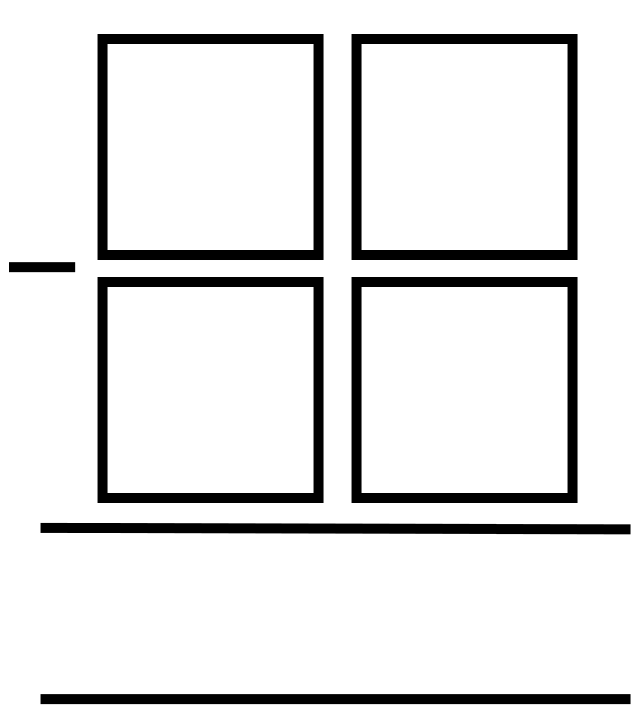#### You may also like### Consecutive Numbers

An investigation involving adding and subtracting sets of consecutive numbers. Lots to find out, lots to explore.### Calendar Capers

Choose any three by three square of dates on a calendar page...Make a set of numbers that use all the digits from 1 to 9, once and once only. Add them up. The result is divisible by 9. Add each of the digits in the new number. What is their sum? Now try some other possibilities for yourself!

# Making a Difference

##### Age 11 to 14Challenge Level

There are a number of ways the digits $2, 5, 7, 8$ can be placed in a subtraction sum like the one below:In this example, the answer is 29.

Can you rearrange the four digits to find all the (positive) answers it is possible to make?Here are two follow-up questions you might like to consider:

• Can you work out which four digits you need to start with to be able to get all the possible answers $7, 9, 11, 13, 18, 22, 29$ and $31$?

• Can you show that, if we're only allowed to use consecutive digits (e.g. $5, 6, 7, 8$), $31$ is the largest possible answer and $7$ is the smallest?

With thanks to Don Steward, whose ideas formed the basis of this problem.